• 拉普拉斯算子是二阶微分，由于图像是坐标系中离散的点，微分方程如下： 对应的算子矩阵如下： 那就尝试一下，cv库有自带的拉普拉斯函数 cv2.Laplacian，此处自写，加强理解。 import numpy import cv2 def ...
两年的研究僧图像处理经验告诉我，微分锐化，积分平滑，至于为什么，仔细想想微分和积分的曲线图就知道了。

拉普拉斯算子是二阶微分，由于图像是坐标系中离散的点，微分方程如下：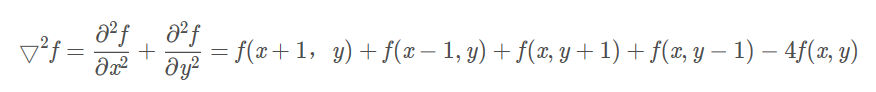二阶微分推导过程如下：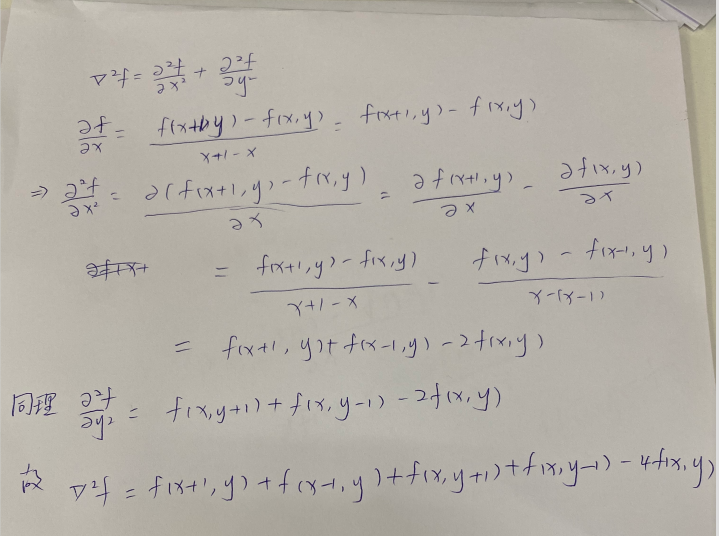对应的算子矩阵如下：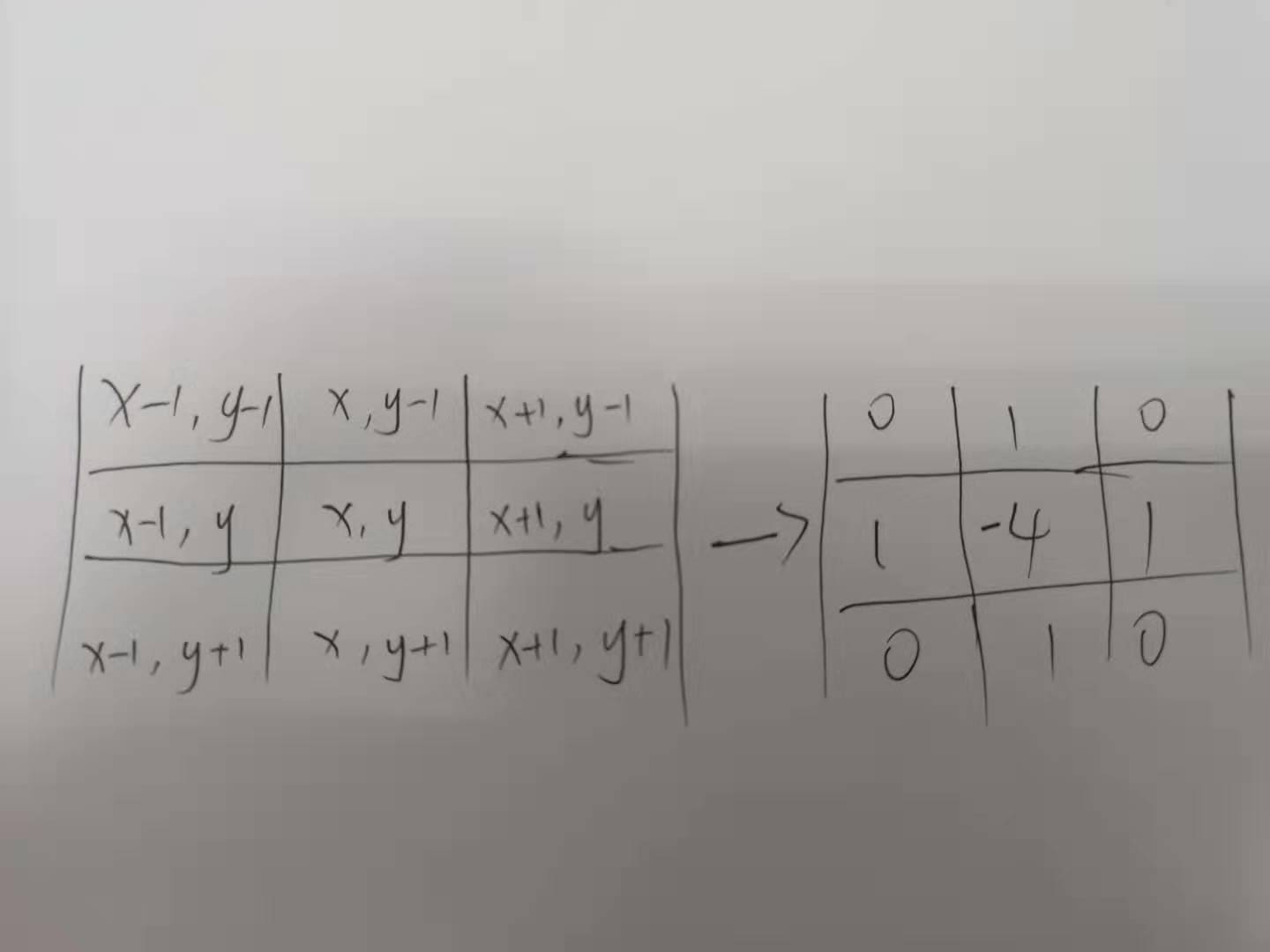那就尝试一下，cv库有自带的拉普拉斯函数 cv2.Laplacian，此处自写，加强理解。

import numpy
import cv2

def laplace2(img):
g = numpy.array(((0, 1, 0), (1, -4, 1), (0, 1, 0)))
# g = numpy.array(((1, 1, 1), (1, -8, 1), (1, 1, 1)))
re = numpy.zeros_like(img)    #生成与img相同shape的全0数组
for i in range(1, img.shape - 1):
for j in range(1, img.shape - 1):
re[i, j] = (img[i-1 : i+2, j-1 : j+2] * g).sum()#+img[i,j]
cv2.imwrite('./'+'1.jpg',re)
return re

img=cv2.imread('demo.jpg')
img=cv2.cvtColor(img,cv2.COLOR_BGR2GRAY)
laplace2(img)

背景噪声还是很多的，所以通常先用高斯滤波消除噪声，本博客只是一个简单粗暴的实验，图片禁止盗取~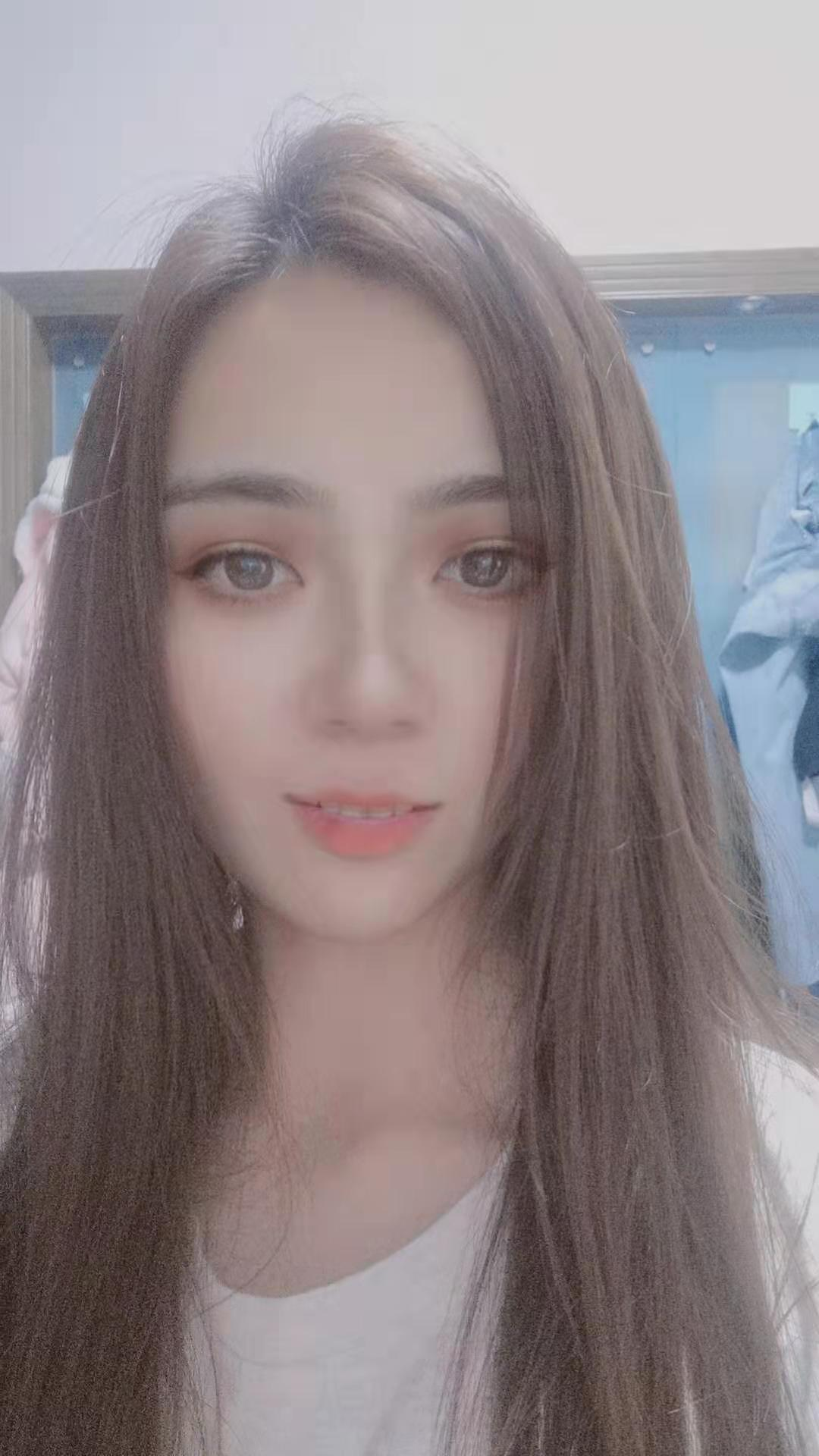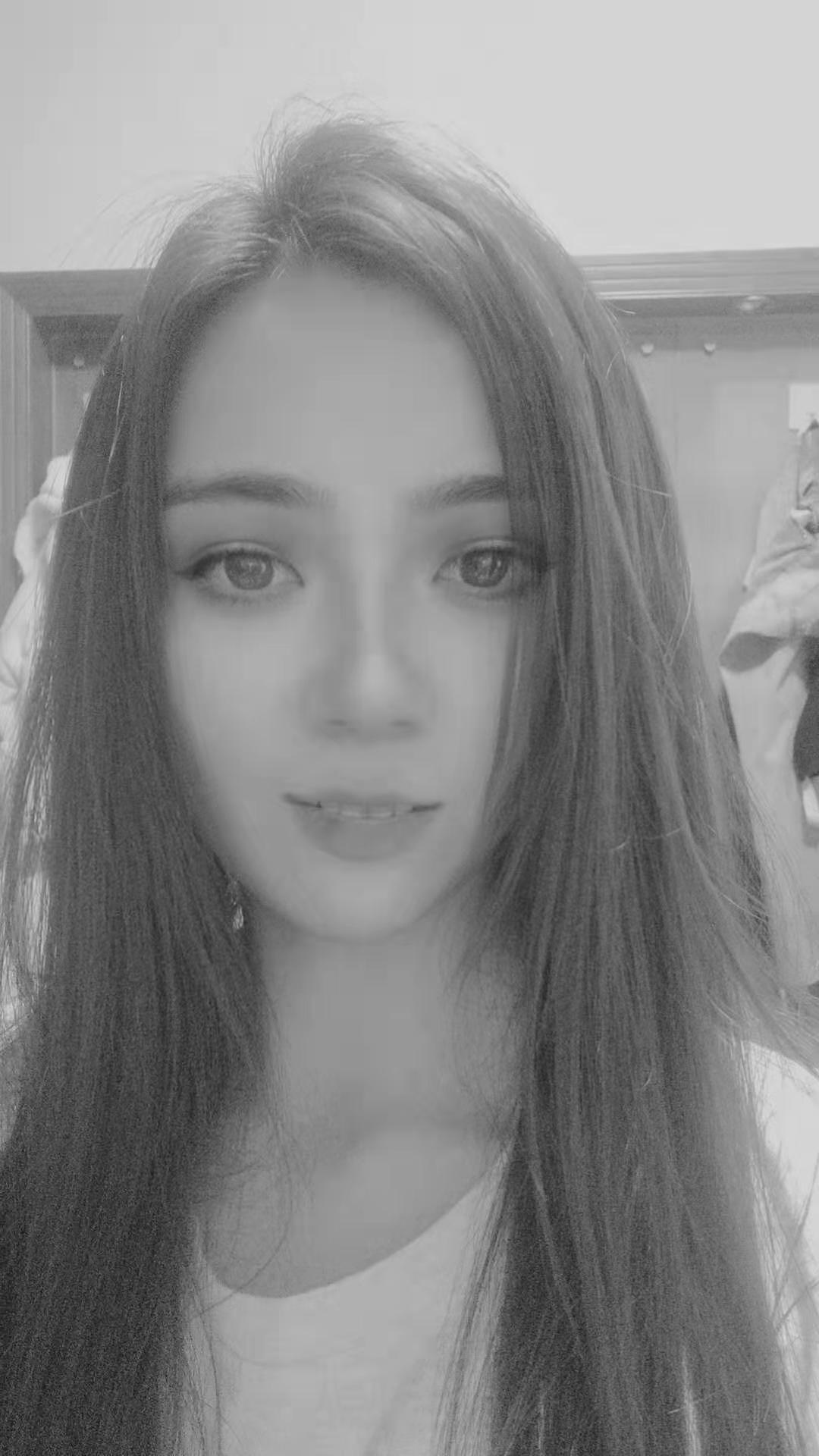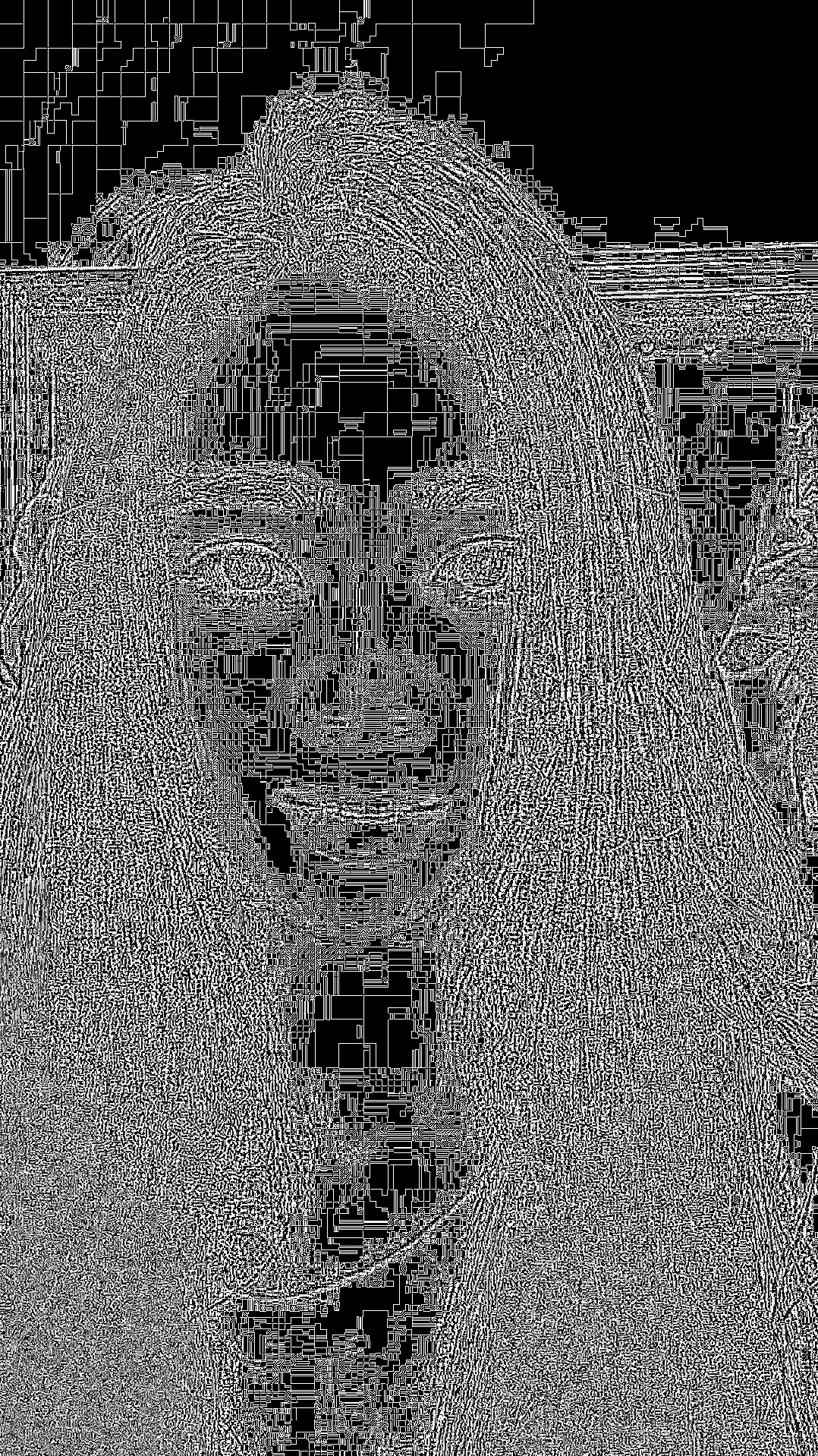由此延伸，拉普拉斯算子是图像的二阶导，可以检测图像灰度值的快速变化，拉普拉斯算子经常用于图像的边缘检测。正常图像中边界清晰，经拉普拉斯计算后方差较大；模糊图像边界信息少，方差小。所以，可以利用拉普拉斯算子做图像的模糊检测。

一句代码搞定，cv2.Laplacian(image, cv2.CV_64F).var()。然后根据样本设置合适的阈值。


展开全文图像处理
• 大致思路如下 对图片做降噪滤波处理 ...使用canny算子进行边缘查找，并使用findContours方法提取边缘坐标点。 使用boundingRect方法找出硬币所在的矩形进行裁切，使用minEnclosingCircle找出包络圆作为蒙...
大致思路如下

对图片做降噪滤波处理
寻找硬币的边缘
使用硬币边缘打造蒙版，并切割出图像

对应的方法如下

对图片进行二值化COLOR_BGR2GRAY处理，高斯滤波GaussianBlur处理。
使用canny算子进行边缘查找，并使用findContours方法提取边缘坐标点。
使用boundingRect方法找出硬币所在的矩形进行裁切，使用minEnclosingCircle找出包络圆作为蒙版，并使用bitwise_and隐藏背景。

首先导入必要的库，测试一下是否加载成功，注意cv2.waitKey(0)一定不要忘记！
import cv2
import numpy as np

image = cv2.imread("coins.png")
cv2.imshow("if imread successfully",image)
cv2.waitKey(0)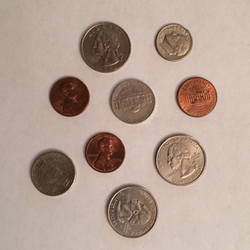接着进行灰度处理和模糊处理，在这之前需要关掉第一张图像
gray = cv2.cvtColor(image,cv2.COLOR_BGR2GRAY)
blurred = cv2.GaussianBlur(gray,(11,11),0)
cv2.imshow("blurred",blurred)
cv2.waitKey(0)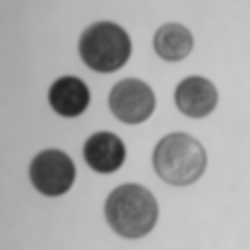进行边缘提取
edged = cv2.Canny(blurred, 30, 150)
cv2.imshow("edged",edged)
cv2.waitKey(0)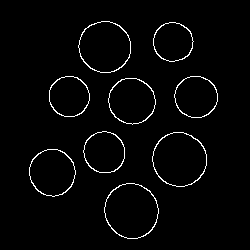在原图中进行边缘提取
# 注意这里只能返回2个变量
(cnts,_) = cv2.findContours(edged.copy(),cv2.RETR_EXTERNAL,cv2.CHAIN_APPROX_SIMPLE)
print(" {} coins in the image".format(len(cnts)))

 显示：9 coins in the image

然后画在原图中
coins = image.copy()
cv2.drawContours(coins, cnts, -1, (0,255,0), 2)
cv2.imshow("coins",coins)
cv2.waitKey(0)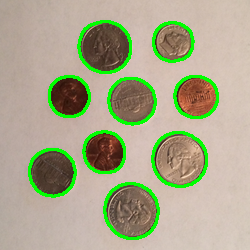最后对图片进行裁切
coins = image.copy()
cv2.drawContours(coins, cnts, -1, (0,255,0), 2)
cv2.imshow("coins",coins)
cv2.waitKey(0)

for (i,circle) in enumerate(cnts):
# circle是每个提取出来的圆
(x,y,w,h) = cv2.boundingRect(circle)
print("coin #{}".format(i+1))
coin_canvas = image[y:y+h, x:x+w]
cv2.imshow("coin",coin_canvas)
cv2.waitKey(0)

mask = np.zeros(image.shape[:2], dtype = 'uint8')
((centerX,centerY),radius) = cv2.minEnclosingCircle(circle)
# 在蒙版上画圆，这里是灰度图，所以255表示白色
cv2.circle(mask,(int(centerX),int(centerY)), int(radius), 255, -1)
mask = mask[y:y+h, x:x+w]
# 自身和自身进行与运算即可
cv2.imshow("masked coin",cv2.bitwise_and(coin_canvas, coin_canvas, mask = mask))
cv2.waitKey(0)

裁切出来的图片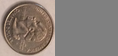加上蒙版的图片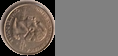以上代码均在notebook中运行通过。


展开全文• 目的 将输入文档使用透视变换将不规则图形变换，...需要变换前pst1和变换后的4个坐标点pst2 使用函数获得M矩阵 pts1 = np.float32([[56,65],[368,52],[28,387],[389,390]]) pts2 = np.float32([[0,0],[300,0],[0,300
目的
将输入文档使用透视变换将不规则图形变换，然后使用tesseract库进行识别文字
变换前图形变换后图形步骤
1.加载原图并显示2.重新调整大小3.灰度处理4.滤波5.边缘检测6.找出轮廓7.透视变换
主要的步骤：
需要变换前pst1和变换后的4个坐标点pst2：这里可以使用图形的4个顶点
使用函数获得M矩阵
pts1 = np.float32([[56,65],[368,52],[28,387],[389,390]])
pts2 = np.float32([[0,0],[300,0],[0,300],[300,300]])
M = cv.getPerspectiveTransform(pts1,pts2)
根据M矩阵获得变换后的图形矩阵
dst = cv.warpPerspective(img,M,(300,300))根据修改好的图形使用tesseract库来识别图中文字代码实现
图形处理模块
import cv2 as cv
import numpy as np
import math
import pytesseract

def resized_img(img, width=None, height=None, inter = cv.INTER_AREA):
"""

等比例重新调整大小
:param img:
:param width: 宽度整数
:param height: 高度整数
:param inter:
:return: 返回调整后的图形矩阵
"""
# 如果宽高都为0
if height is None and width is None:
return img

h,w = img.shape[:2]
# print(w,h)
# 同比例放缩图  形
prop = w/h
# print(prop)

if height is not None and width is not None: # 宽高都给定
return cv.resize(img,(width,height),interpolation=inter)
elif height is not None:# 给定高度计算宽度
w = int(height*prop)
return cv.resize(img,(w,height),interpolation=inter)
else:
h = int(width/prop)# 给定宽度计算高度
# print(h)
return cv.resize(img,(width,h),interpolation=inter)

def show(img, name="image", model=0):
"""

:param img:
:param name:
:param model: 是否可以缩放图片
:return:
"""
if model == 0:
cv.namedWindow(name, cv.WINDOW_NORMAL)
cv.imshow(name, img)
cv.waitKey(0)
cv.destroyAllWindows()

def order_point(contour):
"""
对输入的4个坐标进行排序
分别为左上，右上，右下，左下
:param contour:
:return:
"""
# contour(4,1,2)
print(contour.shape)
print(contour)
rect = np.zeros((4,2), dtype=np.float32)
s = contour.sum(axis=1)
print(s)
sort_s = sorted(s, key=lambda x:x)
left = sort_s[:2]
left_sort = sorted(left, key=lambda x:x)
rect = left_sort
rect = left_sort
right=sort_s[2:4]
right_sort = sorted(right, key=lambda x:x)
rect = right_sort
rect = right_sort
return rect

def new_point(origin):
"""
根据原始的4个点位置得到新的4个对应点的位置

:param origin:
:return:对应宽高 以及目标4个位置
"""
top_left, top_right, bottom_right, bottom_left = origin
# print(top_left,top_right,bottom_right,bottom_left)
# 根据输入的坐标计算新的坐标
w1 = math.sqrt(((top_right-top_left)**2)+((top_right-top_left)**2))
w2 = math.sqrt(((bottom_right-bottom_left)**2)+((bottom_right-bottom_left)**2))
print(w1,w2)
w = int(max(w1, w2))
h1 = math.sqrt(((top_right-bottom_right)**2)+((top_right-bottom_right)**2))
h2 = math.sqrt(((top_left - bottom_left) ** 2) + ((top_left - bottom_left) ** 2))
print(h1,h2)
h = int(max(h1,h2 ))
dst = np.array(
[
[0, 0],
[w - 1, 0],
[w - 1, h - 1],
[0, h - 1],
],dtype=np.float32
)
return (w,h),dst

def ocr_preprocess():
"""对输入图像进行变换成规矩的图形"""
# 加载原彩色图
image = cv.imread("image/ocr_recoginze/page.jpg")
show(image, name="image", model=0)
print(image.shape)

# 对图像缩放用于测试方便观察
ration = image.shape/500
print(ration)
resiz_color_img = resized_img(image, height=500)
show(resiz_color_img, name="resiezed_color_img", model=1)
print(resiz_color_img.shape)

# 原图进行后续操作
# resiz_color_img = image
# 转化为灰度图
gray = cv.cvtColor(resiz_color_img, cv.COLOR_BGR2GRAY)
show(gray, name="gray", model=1)

# 滤波操作
gray = cv.GaussianBlur(gray,(5,5),0)
show(gray, name="gauss", model=1)

# 边缘检测
edge = cv.Canny(gray, 100, 200, apertureSize=3)
print(edge)
show(edge, name="edge", model=1)

# 边缘检测过后已经是二值化的图像
# 进行轮廓提取
contours, hierarchy = cv.findContours(edge, cv.RETR_EXTERNAL, cv.CHAIN_APPROX_SIMPLE)

# 找到最大的5个轮廓
contours = sorted(contours, key=cv.contourArea, reverse=True)[:5]
print(len(contours))

fit_controus = []
# 轮廓近似
for contour in contours:
arclen = cv.arcLength(contour,closed=True)
epsilon = 0.01*arclen
approx = cv.approxPolyDP(contour, epsilon=epsilon, closed=True)
# 轮廓近似为4个点
if len(approx) == 4:
fit_controus.append(approx)
break

# 画出轮廓
cv.drawContours(resiz_color_img, fit_controus, -1 ,(0,255,0),1)
show(resiz_color_img, name="outline", model=1)

# 透视变换
for contour in fit_controus:
# 原始图像4个点的位置
print("原始：",contour)
print("ration后的：",ration*contour)
origin = order_point(contour*ration)
# 对应变换后的4个点的位置
size, dst = new_point(origin)
print(size)
# 获得变换矩阵
perspect_matric = cv.getPerspectiveTransform(origin, dst)
print(perspect_matric)
# 得到变换后的图像矩阵
wraped = cv.warpPerspective(image,M=perspect_matric,dsize=size)
show(wraped, name="wraped")
cv.imwrite("my_page.png", wraped)

if __name__ == '__main__':
ocr_preprocess()


图形识别模块
import pytesseract
from PIL import Image
# fp = open("my_page.png",'br')
text = pytesseract.image_to_string(Image.open("my_page.png"))
print(text)



展开全文opencv 计算机视觉
• 针对自动驾驶系统的实际需求，我们需要一种能够兼顾速度、不同环境下高...通过边缘提取获取特征线，以多约束条件限制获取车道线坐标位置传送给控制单元。由控制单元执行PID算法实时控制车辆根据地面车道线循迹寻找车...
针对自动驾驶系统的实际需求，我们需要一种能够兼顾速度、不同环境下高检测成功率和鲁棒性的车道识别算法。首先，因为停车场更多的是地下光线昏暗的情况需要提高车道线与周围环境的对比度，然后灰度化图像进行模糊降噪处理。因为摄像头相对于车身是固定的所以只需根据特定位置进行处理。通过边缘提取获取特征线，以多约束条件限制获取车道线坐标位置传送给控制单元。由控制单元执行PID算法实时控制车辆根据地面车道线循迹寻找车位。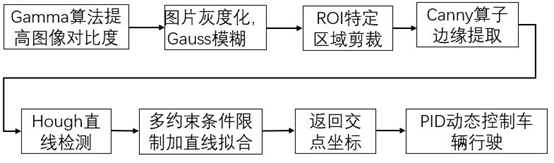Gamma校正
因为人眼对外界光源的感光值与输入光强不是呈线性指数关系的。在低照度下，人眼更容易分辨出亮度的变化，随着照度的增加，人眼不易分辨出亮度的变化。而摄像机感光与输入光强呈线性关系。为了使摄像机能像人眼一样拥有灰暗环境或光线反射较强的情况下颜色的识别度，需要将摄像机采集的图像进行Gamma校正。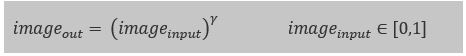当γ小于1时，imageinput越小，增强效果越明显，当γ大于1时，imageinput越大，增强效果越明显。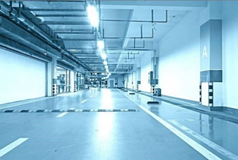图像灰度化并高斯模糊
通常情况下，车道线为白色或黄色线条，与图像中的路面以及其他车辆等背景形成明显差别。为保持R，G，B三通道的平衡性，将灰度值定义为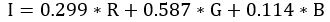得到灰度图片后，因为实际环境并不如理想状态一样，得到的图像拥有很多噪声，我们需要对其进行降噪处理。本文应用的方法是高斯模糊（Gaussian Blur），也叫做高斯平滑。通常用它来减少图像噪声以及降低细节层次，以增强图像在不同比例下的图像效果。高斯模糊是一种图像模糊滤波器，他用正态分布计算图像中每个像素变化。在二维空间定义为：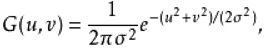效果图如下：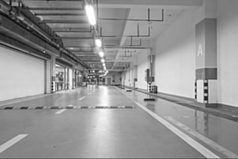因为车载摄像头的位置相对于车辆来说是固定不动的，所以不必对整个图像进行处理。通过ROI剪裁获取图片固定位置的车道线进行处理可以大大降低行车电脑ECU（Electronic Control Unit）的工作负担，提高ECU的处理速度，大大提高车辆控制单元的即时性。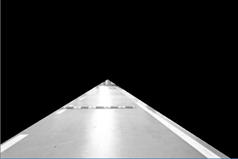Ganny算子的边缘提取
Canny边缘检测算子使John F.Canny于1986年开发出来的一个多级边缘检测算法，它的目标是找到一个最有的边缘检测算法。相较于差分运算法、Sobel、Scharr、Laplace(拉普拉斯)、Roberts边缘算法，Canny算子具有好的信噪比、高的定位新能、对单一边缘仅有唯一响应等优势。算法实现步骤如下：

用高斯滤波器对图像滤波，以出去图像中的噪声。
寻找图像的强度梯度（intensity Gradients）。
应用非最大抑制（non-maximum suppression）技术来消除边误检。
应用双阈值的方法来决定可能存在的边界。
利用滞后技术来跟踪边界。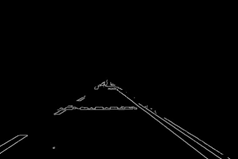基于Hough变换的车道线检测与选取
Hough变换于1962年由Paul Hough提出，并在美国作为专利被发表。它所实现的是一种从图像空间到参数空间的映射关系。由于其根据局部度量来计算全面描述参数，因而对于区域边界被噪声干扰或被其他目标遮盖而引起边界发生某些间断的情况，它具有很好的容错性和鲁棒性。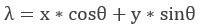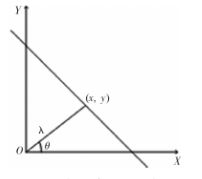其中，λ：为该直线到原点的距离；θ：为过原点到该直线的垂线与X轴正向夹角，表面直线方向。相对于原图像空间XY，参数λ和θ构成新的坐标系为参数空间。原图像空间中的每个点，对应于新参数空间中的一条正弦曲线；原图像中的一条直线，对应参数空间中所有的正弦曲线经过同一点。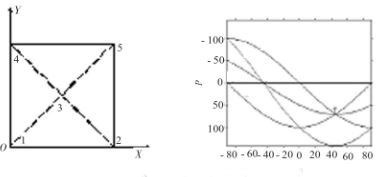图像空间中2、3、4的所对应的参数空间正弦曲线交于T点，表明点2、3、4处在同一直线上。
为了能从Hough检测所获得的直线簇中，获取正确的车道线，需要结合车道线的特征。车道线倾斜角度θ相对于车辆固定可知：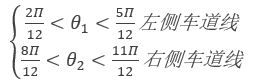因为本文需要的是两条车道线，对满足上述条件的的直线仍然需要进行直线拟合处理，本文采用最小二乘法多项式直线拟合，根据得到满足要求的直线，求近似直线，使得近似直线与各个直线的偏差最小。对应得到两条当前车道线并延长，然后得到其交点坐标。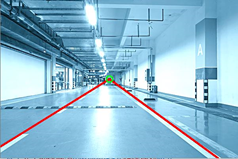原理如上，明天再对代码进行详解。纯属个人见解，如若有误欢迎交流。


展开全文opencv 车道检测
• 通过边缘检测，得到每个工件的坐标，并计算出工件的中心来标记工件的ID 通过工件的每一帧位移量来确定是否为同一个工件 将每一个工件截取出来，进行缺陷的提取提取的缺陷进行直方图计算，通过直方图来值归一化，...
• 宽度检测： 在传统的自动化生产中，对于尺寸的...步骤是先选取出一个矩形，然后进行阈值分割，再进行反色，边缘提取之后进行点的选择，输出坐标做出两条线段，根据线段进行矩形绘制，这样之后就可以计算两条直线之间# python边缘坐标提取python 订阅